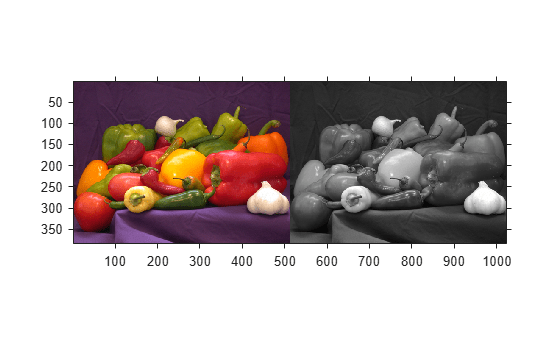# imapplymatrix

Linear combination of color channels

## Syntax

``Y = imapplymatrix(M,X)``
``Y = imapplymatrix(M,X,C)``
``Y = imapplymatrix(___,output_type)``

## Description

````Y = imapplymatrix(M,X)` computes the linear combination of the rows of `M` with the color channels of `X`.```
````Y = imapplymatrix(M,X,C)` computes the linear combination of the rows of `M` with the color channels of `X`, adding the corresponding constant value `C` to each combination.```

example

````Y = imapplymatrix(___,output_type)` returns the result of the linear combination in an array of type `output_type`.```

## Examples

collapse all

This example shows how to create a grayscale image by computing the linear combination of three colors channels.

Read a truecolor image into the workspace.

`RGB = imread('peppers.png');`

Create a coefficient matrix

`M = [0.30, 0.59, 0.11];`

Compute the linear combination of the RGB channels using the coefficient matrix.

`gray = imapplymatrix(M, RGB);`

Display the original image and the grayscale conversion.

`imshowpair(RGB,gray,'montage')`## Input Arguments

collapse all

Weighting coefficients for each color channel, specified as a q-by-p numeric array. p is the length of the third dimension of `X`. In other words, `p=size(X,3)`. q is in the range [1,p].

Input image, specified as an m-by-n-by-p numeric array.

Constant to add to each channel during the linear combination, specified as q-element numeric vector, where q is the number of rows in `M`.

Data Types: `double`

Output data type, specified as one of the following: `'double'`, `'single'`, `'uint8'`, `'uint16'`, `'uint32'`, `'int8'`, `'int16'`, or `'int32'`.

Data Types: `char` | `string`

## Output Arguments

collapse all

Output image comprised of the linear combination of the rows of `M` with the color channels of `X`, returned as a numeric array. If `output_type` is not specified, the data type of `Y` is the same as the data type of `X`.

## Version History

Introduced in R2011b

expand all